# SQL Server IF…ELSE Statement: T-SQL Example

## SQL Server IF…ELSE Statement: T-SQL Example

#### Why do you need Conditional Statements?

In real life, you perform many actions which are dependent on the outcome of some other activity or situation.

Some real-time examples are:

• If it rains tomorrow, I will plan on a road trip.
• If flight tickets are less than \$400 from my city, then I will go on vacation in Europe, else I will prefer some nearby tourist spot.

Here, you can see that one action, like Road trip above, is conditionally dependent on the outcome of another activity which is “whether it will rain or not tomorrow!”

Similarly, MS SQL also provides the capability to execute T-SQL statement conditionally.

In this tutorial, you will learn:

## IF… Else statement

In MS SQL, IF…ELSE is a type of Conditional statement.

Any T-SQL statement can be executed conditionally using IF… ELSE.

Below figure explain IF…ELSE statement

• If the condition evaluates to True, then T-SQL statements followed by IF keyword will be executed.
• If the condition evaluates to False, then T-SQL statements followed by ELSE keyword will be executed.
• Once, either IF T-SQL statements or ELSE T-SQL statement is executed then other unconditional T-SQL statements continues execution.

### Syntax and rules

Syntax:

```IF <Condition>
{Statement | Block_of_statement}
[ ELSE
{Statement | Block_of_statement}]
```

Rules:

• The condition should be Boolean Expression, i.e., condition results in Boolean value when evaluated.
• IF…ELSE can conditionally handle a single T-SQL statement or block of T-SQL statements.
• Block of statement should start with keyword BEGIN and close with keyword END.
• Using BEGIN and END helps SQL server to identify statement block that needs to be executed and separate it from rest of the T-SQL statements which are not part of IF…ELSE T-SQL block.
• ELSE is optional.

### IF…ELSE with the only numeric value in Boolean Expression.

Condition: TRUE

```IF (1=1)
PRINT 'IF STATEMENT: CONDITION IS TRUE'
ELSE
PRINT 'ELSE STATEMENT: CONDITION IS FALSE'
```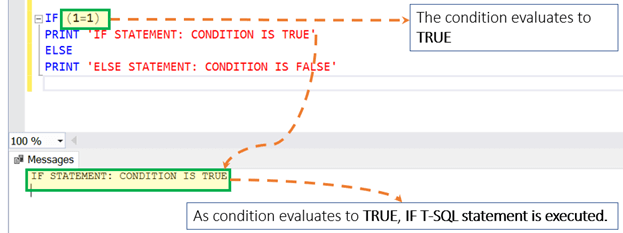Condition: FLASE

```IF (1=2)
PRINT 'IF STATEMENT: CONDITION IS TRUE'
ELSE
PRINT 'ELSE STATEMENT: CONDITION IS FALSE'
```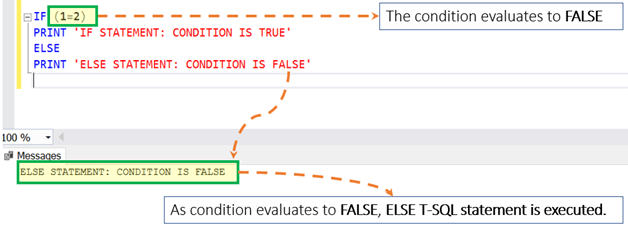Assumption: Assume that you have the table as ‘Guru99’ with two columns and four rows as displayed below: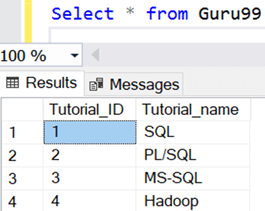We will use ‘Guru99’ table in further examples

### IF…ELSE with the variable in Boolean Expression.

Condition: TRUE

```DECLARE @Course_ID INT = 4

IF (@Course_ID = 4)
Select * from Guru99 where Tutorial_ID = 4
ELSE
Select * from Guru99 where Tutorial_ID != 4
```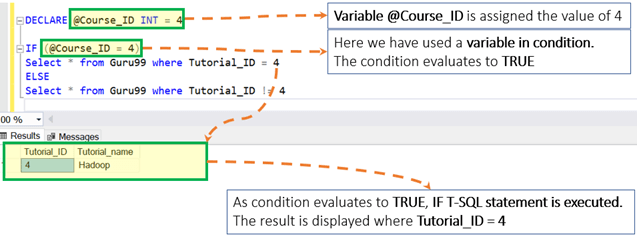Condition: FLASE

```DECLARE @Course_ID INT = 4

IF (@Course_ID != 4)
Select * from Guru99 where Tutorial_ID = 4
ELSE
Select * from Guru99 where Tutorial_ID != 4
```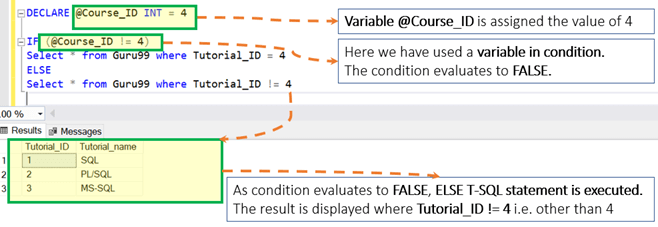### IF…ELSE with Begin End

Condition: TRUE

```DECLARE @Course_ID INT = 2

IF (@Course_ID <=2)
BEGIN
Select * from Guru99 where Tutorial_ID = 1
Select * from Guru99 where Tutorial_ID = 2
END
ELSE
BEGIN
Select * from Guru99 where Tutorial_ID = 3
Select * from Guru99 where Tutorial_ID = 4
END
```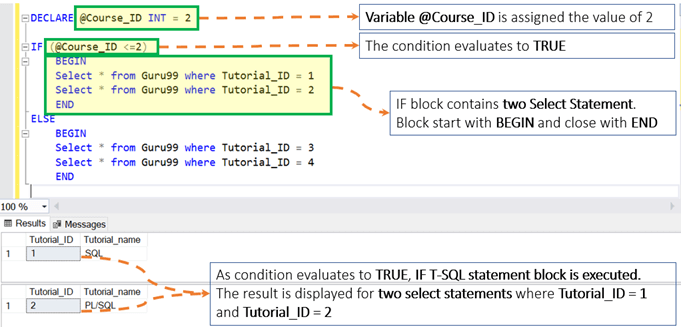Condition: FALSE

```DECLARE @Course_ID INT = 2

IF (@Course_ID >=3)
BEGIN
Select * from Guru99 where Tutorial_ID = 1
Select * from Guru99 where Tutorial_ID = 2
END
ELSE
BEGIN
Select * from Guru99 where Tutorial_ID = 3
Select * from Guru99 where Tutorial_ID = 4
END
```## IF statement with No Else

You can use an IF statement without an ELSE part. Remember we stated that the ELSE part is optional. For example:

```DECLARE @Course_ID INT = 2

IF (@Course_ID <=2)
Select * from Guru99 where Tutorial_ID = 1
```

It prints the following:Executing the false condition will give no output. Consider the following query

```DECLARE @Course_ID INT = 2

IF (@Course_ID <=0)
Select * from Guru99 where Tutorial_ID = 1
```

The result it## Nested IF…Else Statements

Unlike other programming languages, you cannot add an ELSE IF statement within an IF…ELSE statement. This is why you can nest IF…ELSE statements. It is demonstrated below:

``` DECLARE @age INT;
SET @age = 60;

IF @age < 18
PRINT 'underage';
ELSE
BEGIN
IF @age < 50
PRINT 'You are below 50';
ELSE
PRINT 'Senior';
END;```
• In this example, the code will print underage if the value of @age is below 18.
• If not, the ELSE part will be executed. The ElSE part has a nested IF…ELSE.
• If the value of @age is below 50, this will print You are below 50. If none of these conditions is true, the code will print Senior.## Summary:

• Variables are the object which acts as a placeholder.
• Block of statement should start with keyword BEGIN and close with keyword END.
• Else is optional to use in IF… ELSE statement
• Nesting IF…ELSE in another IF…ELSE statement is also possible.